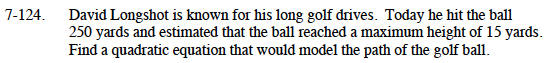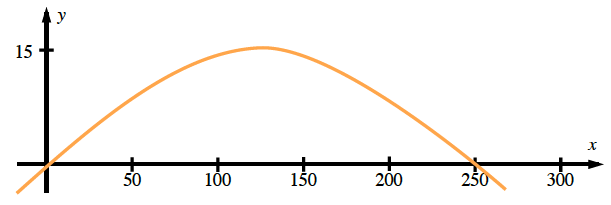Home > CCA2 > Chapter 7 > Lesson 7.2.1 > Problem7-124

7-124.

David Longshot is known for his long golf drives. Today he hit the ball 250 yards and estimated that the ball reached a maximum height of 15 yards. Find a quadratic equation that would model the path of the golf ball. Homework Help ✎A sketch of the graph would look like the one below.You must find the value of a for the quadratic equation.
y = a(xh)2 + k
What is the vertex?
Use the vertex and another point to solve for a.